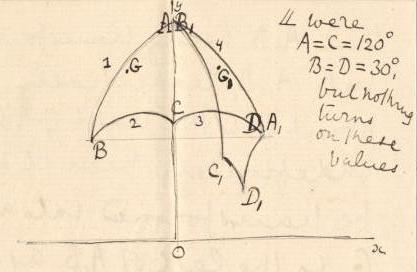## 4-18-2. Arthur Cayley to H. Poincaré

Cambridge 3rd March 1884

Dear Sir,

I have to thank you very much as well for the last paper in the Acta as for the very interesting Notice of your Scientific works.11endnote: 1 Poincaré (1882, 1884). I am at last at leisure to do so, and I am beginning to study your beautiful theory. But I am puzzled with your example I, of the quadrilateral (14, 23).22endnote: 2 Poincaré (1882, § 7). Taking a symmetrical form $ABCD$ as shown in the figure, and applying to it the transformation which converts $AB$ into $DA$, I obtain a new form $A_{1}B_{1}C_{1}D_{1}$ lying on the wrong side of $AD$, and so covering a portion of the area of $ABCD$.Taking $(0,\alpha)(-\ell,m)$ and $(\ell,m)$ for the coordinates of $A$, $B$, $D$ respectively, the formula of transformation is

 $\frac{z_{1}-\alpha i}{z_{1}-\ell-mi}=\frac{\alpha}{m}\frac{z+\ell-mi}{z-\alpha},$

or what is the same thing

 $z_{1}-\frac{\alpha\ell}{\alpha-m}=\frac{-\frac{m\alpha}{(\alpha-m)^{2}}\{\ell^% {2}+(\alpha-m)^{2}\}}{z+\frac{\alpha\ell}{\alpha-m}},$

leading at once to a geometrical construction, which is what I in fact made use in drawing the figure: but I think one sees directly, that $A$, $B$ being transformed into $A_{1}$, $B_{1}$ respectively, a point $G$, to the right of $AB$ & indefinitely near it will be transformed into a point $G_{1}$ to the left of $A_{1}B_{1}$ as shown in the figure. I have ventured to trouble you with this difficulty & I shall be much obliged if you will clear it up for me.33endnote: 3 Poincaré responded the following day; see (§ 4-18-3). I remain dear Sir, yours very sincerely.

A. Cayley

ALS 3p. Private collection, Paris 75017.

Time-stamp: "14.11.2022 20:09"

## References

• H. Poincaré (1882) Théorie des groupes fuchsiens. Acta mathematica 1, pp. 1–62. Cited by: endnote 1, endnote 2.
• H. Poincaré (1884) Notice sur les travaux scientifiques de M. Poincaré. Gauthier-Villars, Paris. Cited by: endnote 1.